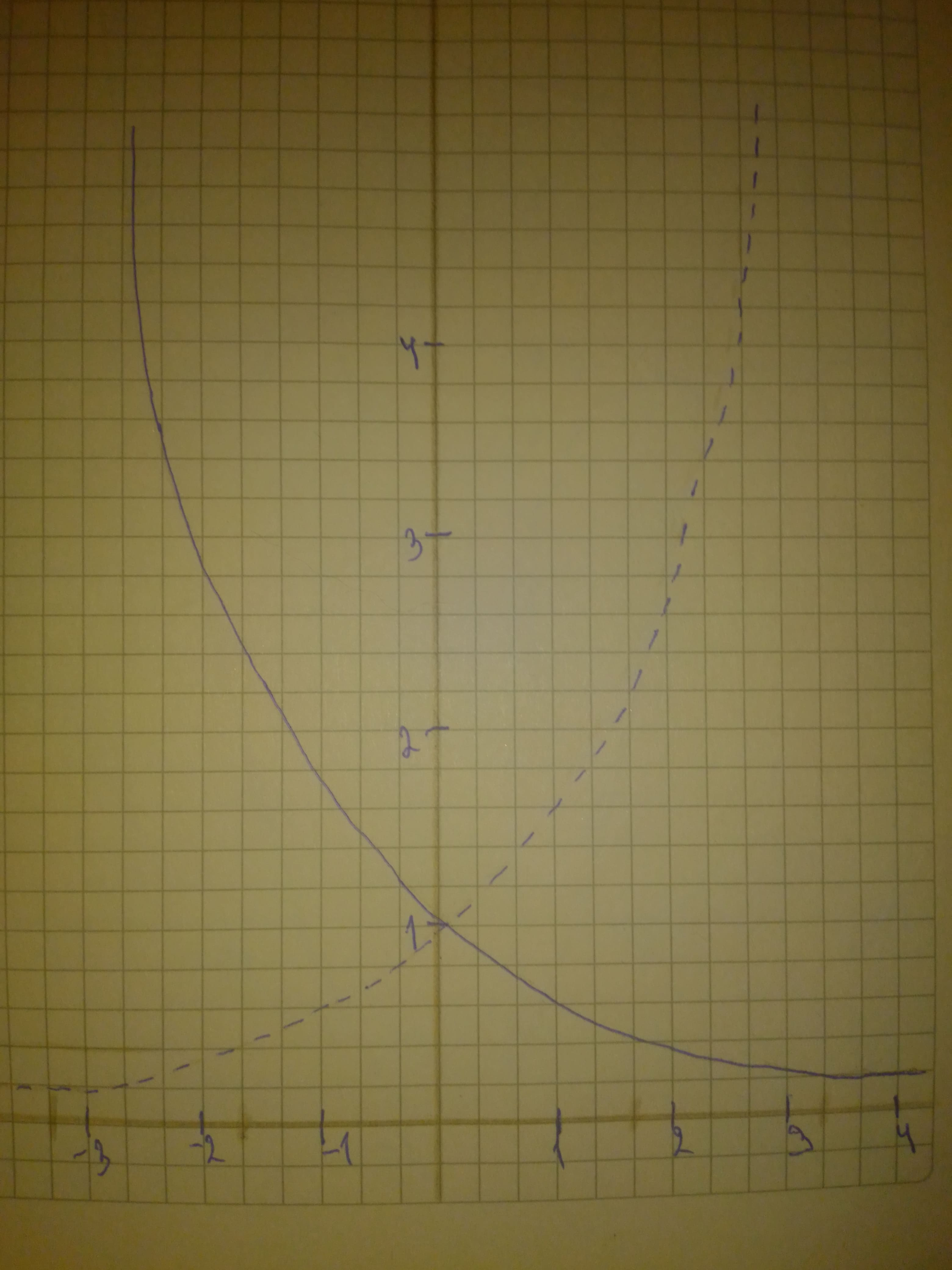# Begin by graphing f(x)=2^{2} Then use transformations of this graph to graph the given function. Be sure to graph and give equations of the asymptotes. Use the graphs to determine each function's domain and range. If applicable, use a graphing utility to confirm your hand-drawn graphs. g(x)=2^{-x}vazelinahS 2021-01-15 Answered
Begin by graphing
$f\left(x\right)={2}^{2}$
Then use transformations of this graph to graph the given function. Be sure to graph and give equations of the asymptotes. Use the graphs to determine each functions
You can still ask an expert for help

• Questions are typically answered in as fast as 30 minutes

Solve your problem for the price of one coffee

• Math expert for every subject
• Pay only if we can solve itBenedict

Step 1Step 2
Graph of ${2}^{x}$ is shown as a dotted curve.
The graph of ${2}^{-x}$ is the graph of ${2}^{x}$ reflected in the y-axis.
Its asymptote has equation $y=0$